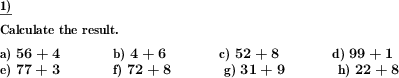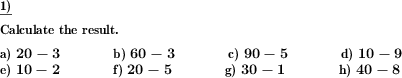Custom math worksheets at your fingertips# Details for problem "Add and subtract sibling numbers"

Quickname: 8802

Elementary School, Primary School.

## Summary

Add and sub sibling numbers in the range from 1 to 100

## Examples## Description

Sibling numbers are numbers whose sum is ten.

This task is used to train sibling numbers that add up to ten.

For this purpose, problems are presented where a two-digit number and a one-digit number must be added or subtracted. With addition, the next tenner is reached, with subtraction the first number is itself a tenner.

It is selectable whether all numbers (1-9) or only a certain number is to be trained. In addition, a trick question can be scattered where the above conditions do not apply.

Download free printable worksheets for this math problem here. The worksheet contains the problems only, the solution sheet includes the answers. Just click on the respective link.

•Worksheet 1Solution sheet with answers
•Worksheet 2Solution sheet with answers
•Worksheet 3Solution sheet with answers

If you can not see the solution sheets for download, they may be filtered out by an ad blocker that you may have installed. If this is the case, please allow ads for this page and reload the page. The solution sheets will then reappear.

• Do these sample worksheets do not really fit?
• Do you need more math worksheets, with a different level of difficulty?
• Would you like to combine different problems on a worksheet and adjust them to your needs?
• As a teacher, you can put together your own worksheets using the automatically generated math problems provided.
With a free initial credit, you can start creating your own math worksheets in a few minutes.

You can try it for free! Register here, to create custom worksheets now!

## Customization options for this problem

Parameter
Possible values
Number of problems
1, 2, 3, 4, 5, 6, 7, 8, 9, 10
Number to practice
1-9, 1, 2, 3, 4, 5, 6, 7, 8, 9
Plus/minus
Mixed, Plus, Minus
Trick question
Yes, No